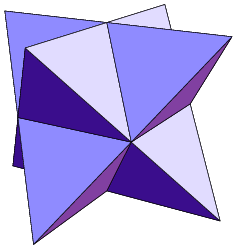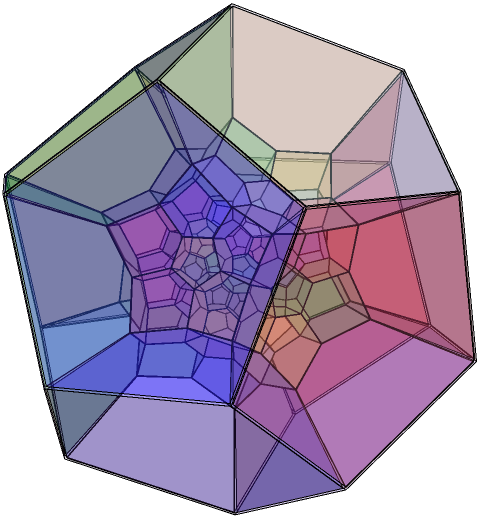# Spin groups

If you take an object and rotate it by 360° about a particular axis, it will return to its original orientation. Certainly, every object you’re likely to have seen obeys this basic principal. If you’re naive, you may actually believe that this applies to everything — an understandable mistake.Consider this perfectly innocent-looking Möbius strip. I’m going to assume without loss of generality that you understand the properties of Möbius strips. You’ll know, therefore, that it only has one side of ‘length’ 720°, as opposed to two sides of length 360°.

As well as being the double-cover of SO(2), spin(2) is isomorphic to SO(2) (the rotations of a circle). This means I can tear along the lateral line of the Möbius strip to turn it into an ordinary band. It’s also isomorphic to U(1), the group of complex numbers of unit modulus.

### SO(3) and Spin(3)

Things become more interesting in three dimensions. We have SO(3), which are the familiar rotations of a sphere. It has three interesting finite subgroups, namely the rotations of an icosahedron, the rotations of a cube and the rotations of a tetrahedron. Any other finite subgroup of SO(3) is boring.

We can describe a rotation by a vector (x,y,z) on its axis and a magnitude w = 2 arccos(θ), where θ is the angle of rotation. Scaling the vector such that w² + x² + y² + z² = 1, we obtain a mapping between the rotation and a quaternion w + xi + yj + zk. It is of great importance that this is a homomorphism from the group of unit quaternions to the group of rotations.

Note that the quaternions q and −q represent the same rotation. In other words, the group of quaternions is isomorphic to the double cover Spin(3) of the rotation group SO(3). The ‘interesting’ subgroups of SO(3) correspond to interesting subgroups of Spin(3):

• The binary tetrahedral group ‘2T’ is the group of 24 Hurwitz units under multiplication. This is the double cover of the rotations of the tetrahedron, which has order 12. There is a normal subgroup of order 8, which you may know as Q8.
• The binary octahedral group ‘2O’ is obtained by adjoining (1 + i)/sqrt(2) to the previous group, to get a group of 48 unit quaternions — the double cover of the rotations of a cube. They can be categorised as ‘even’ (if belonging to 2T) or ‘odd’ (otherwise), with the even elements forming a normal subgroup.
• The binary icosahedral group is the most interesting of all, containing the 120 unit icosians. It is not to be confused with C2 × A5 (the rotational and reflectional symmetries of a dodecahedron) or S5 (permutations of five objects), both of which also have order 120.

Electrons also have to rotate through 720° to return to their original orientations, so their orientations belong to Spin(3) rather than SO(3). An immediate consequence of this is that Hunter Spink can balance a beaker of water on the palm of his hand and rotate his arm through 720°, returning it to its original position.

### SO(4) and Spin(4)

Let l and r be two quaternions of unit length. A mapping q → lqr* is a rotation in four-dimensional space; moreover, all rotations in SO(4) can be represented by such a pair of quaternions (l,r). Note also that (-l,-r) represents the same rotation, so we have the marvellous isomorphism Spin(3) × Spin(3) = Spin(4), which is really amazing.

We should be able to find double covers of the rotational symmetry groups of the Platonic hypersolids. We’ll start with the most unusual one, the 24-cell. Before we get to that, however, it is useful to consider a three-dimensional analogy:

The tetrahedron is self-dual, which means that a greater symmetry group can be obtained by composing it with its dual to form a stella octangula:There is an analogy in four dimensions, where the 24-cell and its dual can be composed to yield an object with a rotational symmetry group of order 1152 instead of 576. The double cover of this, with order 2304, is obtained by choosing any ordered pair (l,r) of quaternions from the binary octahedral group 2O.

Recall that 2O has 2T as a normal subgroup, allowing us to refer meaningfully to ‘odd’ and ‘even’ rotations. If we add the additional constraint that l and r have the same parity, we get down to a group of order 1152, the double cover of the symmetry group of the 24-cell.

Going even further, note that 2O has Q8 as a normal subgroup of index 6, so we can define a homomorphism θ from 2O to the cyclic group C6, the kernel of which is Q8. Insisting further that θ(l) = θ(r), we get the rotations of the hypercube as a subgroup of the rotations of a 24-cell.The 120-cell (pictured above using stereographic projection) has 7200 rotational symmetries. Its double cover corresponds exactly to 2I × 2I, i.e. the group of ordered pairs (l,r) of unit icosians. Some order-120 index-120 subgroup of this is the double cover of the rotational symmetry group of the 5-cell (four-dimensional simplex), isomorphic to 2I.

In even higher dimensions, Spin(6) is apparently isomorphic to SU(4), the ‘rotations’ of a ‘sphere’ in complex Euclidean 4-space.

This entry was posted in Uncategorized. Bookmark the permalink.

### 4 Responses to Spin groups

1.Anonymous says:

Great article and blog. Sorry, it’s not mentioned in the ‘contact’ or ‘about’ page, are you a Cambridge student?

•apgoucher says:

Thanks. I am indeed; why do you ask? Your IP address suggests that you’re also a Cantabrigian, and possibly from Trinity.

•Anonymous says:

Thanks for the reply. No reason – just curious! Yes, I am a first year student at Cambridge doing Maths – what year are you in?

2.Daniel Sebald says:

Spin(5) is also isomorphic to Sp(2), the ‘rotations’ of a ‘sphere’ in quaternionic Euclidean 2-space.